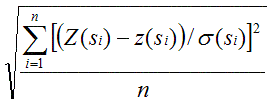HOW TO

# Create a cross validation graph so that the root-mean-square-standardized error is close to 1

## Summary

Creating cross validation graphs show a good fit, but the root-mean-square-standardized error is not close to 1. When many data points are plotted on a graph, the graph may become crowded and interpretations become difficult.

Instructions provided are to create a graph in other spreadsheet packages, such as MS Excel, using the cross validation output created by Geostatistical Analyst.

## Procedure

1. In Geostatistical Analyst, click Save Cross Validation...
The output will be saved as a .dbf table.
2. Plot 'measured' versus 'predicted' values on a graph.
3. Verify that the root mean square standardized error is calculated correctly. Do this by using the following formula:Article ID:000006874

Software:
• ArcMap 8 x
• ArcMap 9 x

Get help from ArcGIS experts

Contact technical support